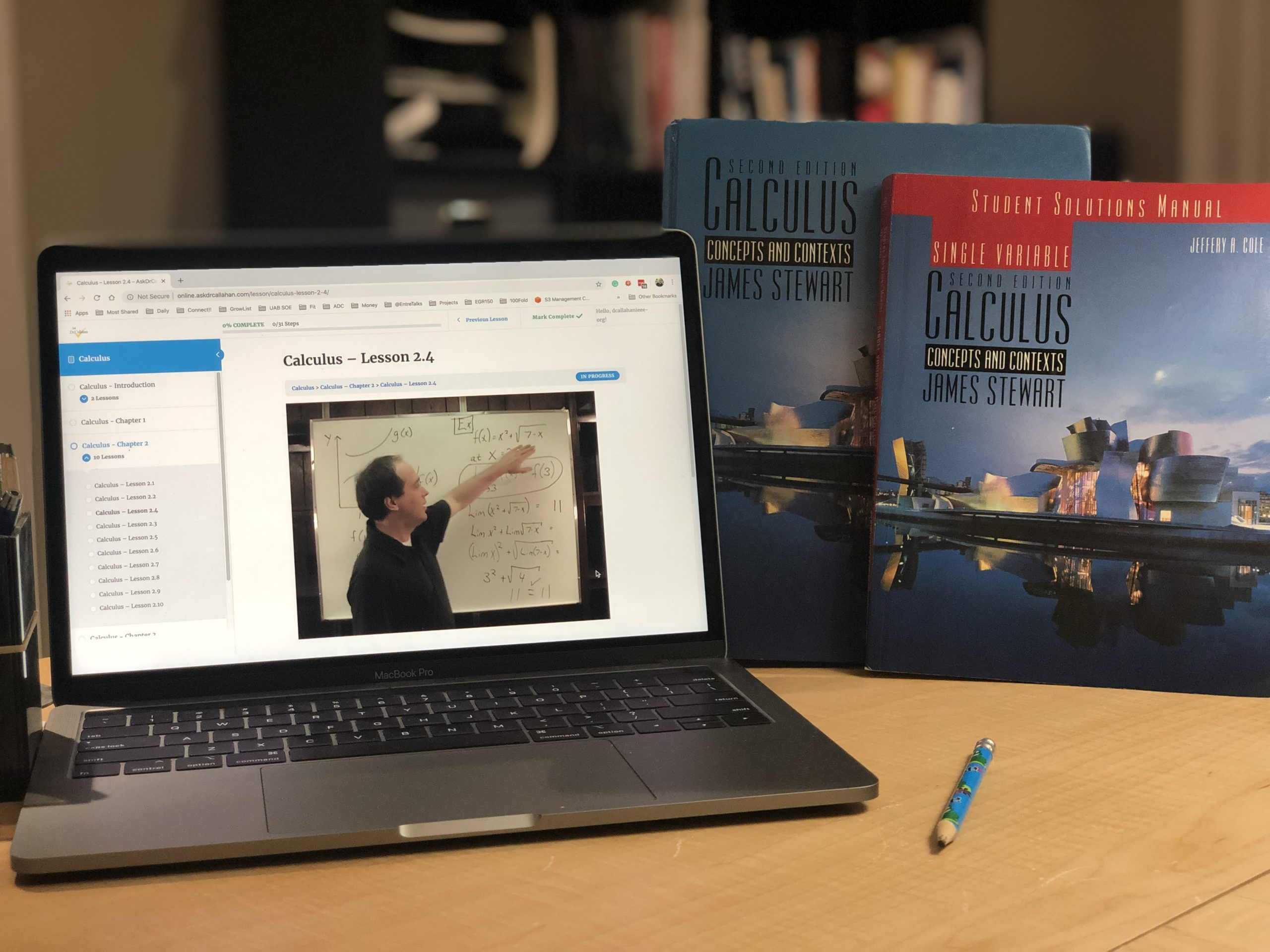Posted on

# Calculus 1 Course Description for TranscriptIf you are looking for details for a transcript for Calculus 1,  this should do the trick.

Calculus is about change. The tools of calculus allow us to model change. Once we have equations for something, we can use calculus to see the impact of change on the system.

In Algebra, we learned how to generalized basic arithmetic to show relationships. So, instead of saying 2 + 2 = 4, we might say 2 + x = y, allowing a more general equation that shows a relationship between x and y.

In Geometry (which also includes trigonometry), we studied the relationships of shape.

In Calculus, we study continuous change. For example, our car gets so much gas milage which is impacted by the car’s weight. But the longer you drive, the less gas you have and therefore the less weight. So the weight of the car changes over time, therefore impacting the use of fuel over time.

Calculus is a necessary tool for any science or engineering. It is heavily used in physics and engineering and found in biology, business, social sciences, etc. No matter what we are studying, the only constant thing is change.

Calculus is broken up into two areas – Derivative and Integral Calculus. This course covers derivative calculus, which is equivalent to most Calculus 1 courses at most universities. Calculus 2 covers integral calculus.

Students who complete Calculus are prepared to enter college and should be able to step into a college-level Calculus 1 or Calculus 2 course – depending on how the individual university defines its course. Calculus is not required for the ACT and the SAT.

Topics Covered in this Course:

• Limits and limit laws
• Continuity
• Derivatives
• Differentiation rules
• Application of differentiation
• Antiderivatives